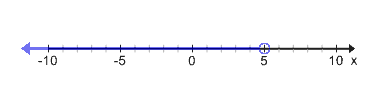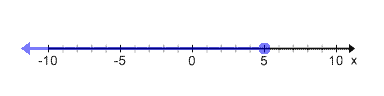In this video, we will be learning how to graph inequalities without using the graphing calculator.

## Example of Graphing Inequalities$x = 5$$x \textless 5$
Here, we include all numbers less than 5 (to the left), but do not shade the circle on 5 because it cannot equal 5.$x \textgreater 5$
Here, we include all numbers greater than 5 (to the right), but do not shade the circle on 5 because it cannot equal 5.$x \leq 5$
We include all numbers less than 5 (to the left) and also shade the circle on 5 because it can equal 5.$x \geq 5$
Here, we include all numbers greater than 5 (to the right) and also shade the circle on 5 because it can equal 5.## Video-Lesson Transcript

Let’s go over how to graph inequalities.

First of all, what is inequality?

Look at this:$x = 5$ is an equation because it is equal to a certain number.

In inequality, it doesn’t exactly equal to.

Examples of inequality are:$x \textless 5$$x \textgreater 5$$x \leq 5$$x \geq 5$

Or could be more complex like this:$x + 7 \textless 5$

So, how do we graph this inequality?

Let’s see how to graph the equation$x = 5$.

Draw a number line. The point that has$5$ is our equation$x = 5$.

Now, let’s look at the inequality$x \textless 5$

Here, we have an infinite number of solutions. Because we can have any number that’s less than$5$.

So,$1, 2, 3, 4$ are all less than$5$.

But so are$4.9, 4.2, -3$.

There are infinite numbers.

Now, let’s graph this.

Draw a number line.

The equation has a point that represents the equals sign.

In inequality, we will just encircle the endpoint. In this case, it’s$5$. Then draw a line going to the left which represents the infinite numbers which are less than$5$.

Let’s have more examples:$x \leq 5$

First, let’s draw a number line.$x$ can be equal to$5$ so we can draw a point on it. Then let’s draw a line to the left which represents all numbers less than$5$.

Now, let’s do$x \textgreater 5$.

Draw a number line.

Let’s encircle the number$5$ and draw a line to the right which represents all numbers greater than$5$.$x \geq 5$

Draw a number line.

Highlight the point in number$5$ and draw a line to the right which represents all numbers greater than$5$.

The line below the less or greater than sign means equal to.$x \leq 5$ has the same meaning as$5 \geq x$.Think of the$\textless$ sign as a mouth. It’s going to eat whatever is bigger.

Likewise we can write$x \textless 5$ as$5 \textgreater x$.$x \geq 5$ is also same as$5 \leq 5$.

And$x \textgreater 5$ can also be written as$5 \textless x$

After you finish this lesson, view all of our Algebra 1 lessons and practice problems.